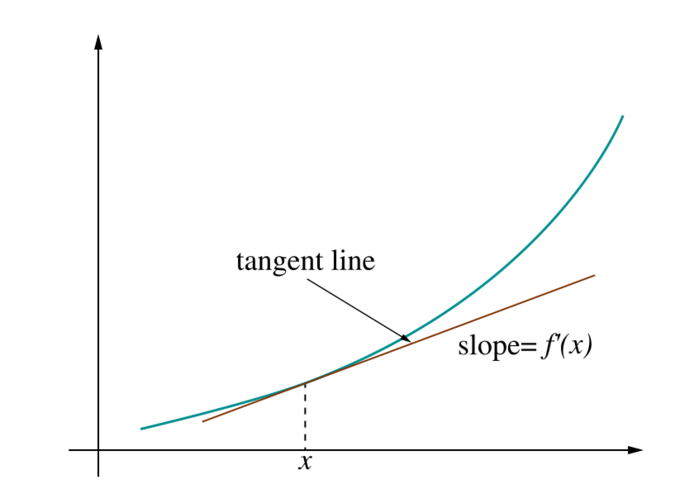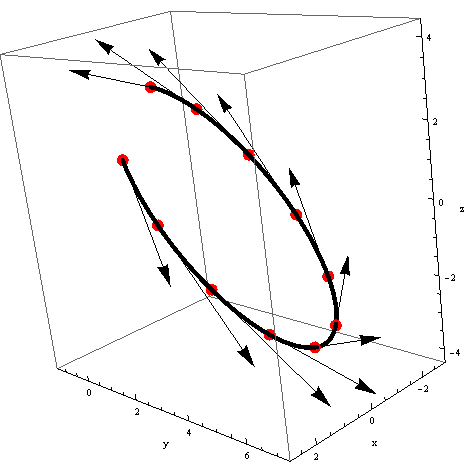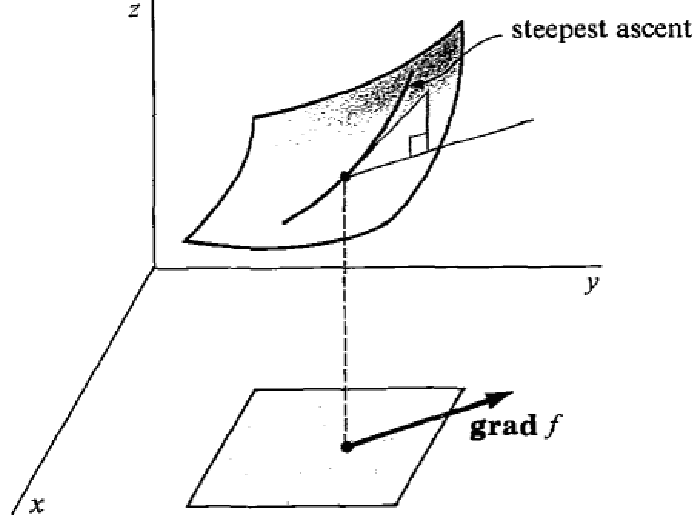# Derivative, Gradient and Jacobian unified

A summary about scalar and vector derivatives.

## Functions of scalars

Let $f: \realset \to \realset$ a function. For $x \in \realset$, the derivative of $f$ at point $x$ is:

This number is the slope of the tangent to the curve described by $f$.## Vector functions of scalars

Let $\vec{f}: \realset \to \realvset{M}$ a function. For $x \in \realset$, the derivative of $\vec{f}$ at point $x$ is:

Which is the vector of the partial derivative:

This vector is tangent to the parametric curve described by $f$.## Functions of vectors

Let $f: \realvset{N} \to \realset$ a function. For $\vec{x} \in \realvset{N}$, the derivative of $f$ at point $\vec{x}$ is:

We call this row vector the gradient of $\vec{f}$. It is noted:

This vector is tangent to the suface described by $f$ and directed along the steepest ascent of $f$.## Vector functions of vectors

Let $\vec{f}: \realvset{N} \to \realvset{M}$ a function. For $\vec{x} \in \realvset{M}$, the derivative of $\vec{f}$ at point $\vec{x}$ can be writen in two equivalent forms.

Since $\frac{\partial}{\partial \vec{x}} f_1(\vec{x})$ is the derivative of a scalar function with respect to a vector, we have:

Which corresponds to the following matrix in $\realvset{N \times M}$:

This matrix is called the jacobian of $\vec{f}$.

Actually, when all the components of $\vec{x}$ are fixed except $x_1$, $\vec{f}(\vec{x})$ can be considered as a function of the scalar $x_1$. In this view, $\frac{\partial}{\partial x_1} \vec{f}(\vec{x})$ is the derivative of a vector function with respect to the scalar $x_1$, and we have:

Other articles you might like: